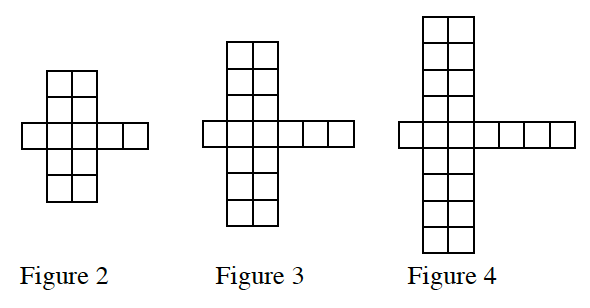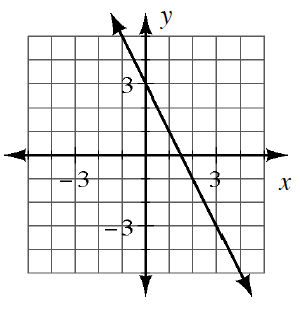### Home > CC4 > Chapter 2 > Lesson 2.2.3 > Problem2-74

2-74.

Each part below represents a different situation. For each situation, determine the pattern of growth and the number of tiles in Figure 0 or the $y$-intercept. Homework Help ✎

1.How many more squares does Figure 3 have than Figure 2?

1.Make a slope triangle between two points on the graph to find the slope, or the growth rate.

Growth rate: $−2$

1. $y=3x−14$

$G = 3, y-int = –14$

2.  $\boldsymbol x$ $-3$ $-2$ $-1$ $0$ $1$ $2$ $3$ $\boldsymbol y$ $18$ $13$ $8$ $3$ $-2$ $-7$ $-12$

What is the difference between each $y$-value?
What is the value of $y$ when $x$ is $0$?

3. Which situation above is growing (increasing) at the fastest rate?